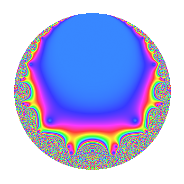# Properties

 Label 27.8.a.eLevel 27 Weight 8 Character orbit 27.a Self dual Yes Analytic conductor 8.434 Analytic rank 0 Dimension 2 CM No Inner twists 1

# Related objects

## Newspace parameters

 Level: $$N$$ = $$27 = 3^{3}$$ Weight: $$k$$ = $$8$$ Character orbit: $$[\chi]$$ = 27.a (trivial)

## Newform invariants

 Self dual: Yes Analytic conductor: $$8.43439568807$$ Analytic rank: $$0$$ Dimension: $$2$$ Coefficient field: $$\Q(\sqrt{65})$$ Coefficient ring: $$\Z[a_1, a_2]$$ Coefficient ring index: $$3$$ Fricke sign: $$1$$ Sato-Tate group: $\mathrm{SU}(2)$

## $q$-expansion

Coefficients of the $$q$$-expansion are expressed in terms of $$\beta = \frac{1}{2}(-1 + 3\sqrt{65})$$. We also show the integral $$q$$-expansion of the trace form.

 $$f(q)$$ $$=$$ $$q + ( 5 + \beta ) q^{2} + ( 43 + 9 \beta ) q^{4} + ( 91 + 2 \beta ) q^{5} + ( 395 + 90 \beta ) q^{7} + ( 889 - 49 \beta ) q^{8} +O(q^{10})$$ $$q + ( 5 + \beta ) q^{2} + ( 43 + 9 \beta ) q^{4} + ( 91 + 2 \beta ) q^{5} + ( 395 + 90 \beta ) q^{7} + ( 889 - 49 \beta ) q^{8} + ( 747 + 99 \beta ) q^{10} + ( 5465 + 40 \beta ) q^{11} + ( -3100 - 720 \beta ) q^{13} + ( 15115 + 755 \beta ) q^{14} + ( -8213 - 459 \beta ) q^{16} + ( 7032 - 2352 \beta ) q^{17} + ( 7418 - 1188 \beta ) q^{19} + ( 6541 + 887 \beta ) q^{20} + ( 33165 + 5625 \beta ) q^{22} + ( 14818 + 5264 \beta ) q^{23} + ( -69260 + 360 \beta ) q^{25} + ( -120620 - 5980 \beta ) q^{26} + ( 135245 + 6615 \beta ) q^{28} + ( -71770 - 260 \beta ) q^{29} + ( -26383 - 14058 \beta ) q^{31} + ( -221871 - 3777 \beta ) q^{32} + ( -308232 - 2376 \beta ) q^{34} + ( 62225 + 8800 \beta ) q^{35} + ( 217010 - 21600 \beta ) q^{37} + ( -136358 + 2666 \beta ) q^{38} + ( 66591 - 2583 \beta ) q^{40} + ( 356650 - 18580 \beta ) q^{41} + ( -532090 + 24660 \beta ) q^{43} + ( 287555 + 50545 \beta ) q^{44} + ( 842634 + 35874 \beta ) q^{46} + ( 762326 - 36848 \beta ) q^{47} + ( 515082 + 63000 \beta ) q^{49} + ( -293740 - 67820 \beta ) q^{50} + ( -1079380 - 52380 \beta ) q^{52} + ( 1319553 + 28638 \beta ) q^{53} + ( 508995 + 14490 \beta ) q^{55} + ( -292705 + 65065 \beta ) q^{56} + ( -396810 - 72810 \beta ) q^{58} + ( 914860 + 97760 \beta ) q^{59} + ( -321688 - 23184 \beta ) q^{61} + ( -2184383 - 82615 \beta ) q^{62} + ( -609533 - 178227 \beta ) q^{64} + ( -492340 - 70280 \beta ) q^{65} + ( 168350 - 9900 \beta ) q^{67} + ( -2788152 - 16680 \beta ) q^{68} + ( 1595925 + 97425 \beta ) q^{70} + ( -2238360 - 234480 \beta ) q^{71} + ( -1473775 + 197640 \beta ) q^{73} + ( -2068550 + 130610 \beta ) q^{74} + ( -1242058 + 26370 \beta ) q^{76} + ( 2684275 + 504050 \beta ) q^{77} + ( 5159384 + 208152 \beta ) q^{79} + ( -881411 - 57277 \beta ) q^{80} + ( -929430 + 282330 \beta ) q^{82} + ( 266657 - 110888 \beta ) q^{83} + ( -46872 - 195264 \beta ) q^{85} + ( 939910 - 433450 \beta ) q^{86} + ( 4572225 - 230265 \beta ) q^{88} + ( 2942790 - 135420 \beta ) q^{89} + ( -10685300 - 498600 \beta ) q^{91} + ( 7554070 + 312338 \beta ) q^{92} + ( -1568178 + 614934 \beta ) q^{94} + ( 328142 - 90896 \beta ) q^{95} + ( 1526255 - 1046160 \beta ) q^{97} + ( 11773410 + 767082 \beta ) q^{98} +O(q^{100})$$ $$\operatorname{Tr}(f)(q)$$ $$=$$ $$2q + 9q^{2} + 77q^{4} + 180q^{5} + 700q^{7} + 1827q^{8} + O(q^{10})$$ $$2q + 9q^{2} + 77q^{4} + 180q^{5} + 700q^{7} + 1827q^{8} + 1395q^{10} + 10890q^{11} - 5480q^{13} + 29475q^{14} - 15967q^{16} + 16416q^{17} + 16024q^{19} + 12195q^{20} + 60705q^{22} + 24372q^{23} - 138880q^{25} - 235260q^{26} + 263875q^{28} - 143280q^{29} - 38708q^{31} - 439965q^{32} - 614088q^{34} + 115650q^{35} + 455620q^{37} - 275382q^{38} + 135765q^{40} + 731880q^{41} - 1088840q^{43} + 524565q^{44} + 1649394q^{46} + 1561500q^{47} + 967164q^{49} - 519660q^{50} - 2106380q^{52} + 2610468q^{53} + 1003500q^{55} - 650475q^{56} - 720810q^{58} + 1731960q^{59} - 620192q^{61} - 4286151q^{62} - 1040839q^{64} - 914400q^{65} + 346600q^{67} - 5559624q^{68} + 3094425q^{70} - 4242240q^{71} - 3145190q^{73} - 4267710q^{74} - 2510486q^{76} + 4864500q^{77} + 10110616q^{79} - 1705545q^{80} - 2141190q^{82} + 644202q^{83} + 101520q^{85} + 2313270q^{86} + 9374715q^{88} + 6021000q^{89} - 20872000q^{91} + 14795802q^{92} - 3751290q^{94} + 747180q^{95} + 4098670q^{97} + 22779738q^{98} + O(q^{100})$$

## Embeddings

For each embedding $$\iota_m$$ of the coefficient field, the values $$\iota_m(a_n)$$ are shown below.

For more information on an embedded modular form you can click on its label.

Label $$\iota_m(\nu)$$ $$a_{2}$$ $$a_{3}$$ $$a_{4}$$ $$a_{5}$$ $$a_{6}$$ $$a_{7}$$ $$a_{8}$$ $$a_{9}$$ $$a_{10}$$
1.1
 −3.53113 4.53113
−7.59339 0 −70.3405 65.8132 0 −738.405 1506.08 0 −499.745
1.2 16.5934 0 147.340 114.187 0 1438.40 320.924 0 1894.75
 $$n$$: e.g. 2-40 or 990-1000 Significant digits: Format: Complex embeddings Normalized embeddings Satake parameters Satake angles

## Inner twists

This newform does not admit any (nontrivial) inner twists.

## Atkin-Lehner signs

$$p$$ Sign
$$3$$ $$1$$

## Hecke kernels

This newform can be constructed as the kernel of the linear operator $$T_{2}^{2} - 9 T_{2} - 126$$ acting on $$S_{8}^{\mathrm{new}}(\Gamma_0(27))$$.